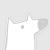### 热门内容082

### 最新内容000

### 最新论文We investigate the adversarial robustness of streaming algorithms. In this context, an algorithm is considered robust if its performance guarantees hold even if the stream is chosen adaptively by an adversary that observes the outputs of the algorithm along the stream and can react in an online manner. While deterministic streaming algorithms are inherently robust, many central problems in the streaming literature do not admit sublinear-space deterministic algorithms; on the other hand, classical space-efficient randomized algorithms for these problems are generally not adversarially robust. This raises the natural question of whether there exist efficient adversarially robust (randomized) streaming algorithms for these problems. In this work, we show that the answer is positive for various important streaming problems in the insertion-only model, including distinct elements and more generally $F_p$-estimation, $F_p$-heavy hitters, entropy estimation, and others. For all of these problems, we develop adversarially robust $(1+\varepsilon)$-approximation algorithms whose required space matches that of the best known non-robust algorithms up to a $\text{poly}(\log n, 1/\varepsilon)$ multiplicative factor (and in some cases even up to a constant factor). Towards this end, we develop several generic tools allowing one to efficiently transform a non-robust streaming algorithm into a robust one in various scenarios.000下载预览
Top